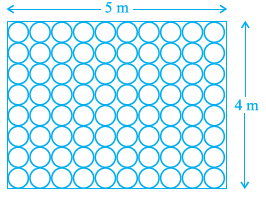#### Floor of a room is of dimensions 5 m × 4 m and it is covered with circular tiles of diameters 50 cm each as shown in Figure. Find the area of floor that remains uncovered with tiles. (Use = 3.14)[4.3 m2]

Solution

Diameter of tile =50cm=0.5m      (1m = 100cm)

Number of tiles lengthwise =  tiles

Number of tiles widthwise =  tiles

Total tiles =

Area of floor not covered by tiles = Area of rectangular floor – Area of 80 tiles